Next: 3.4 Closed Hashing Up: 3.3 Hash Tables Previous: 3.3 Hash Tables

## 3.3.1 Open Hashing

Let:
• U be the universe of keys:
• integers
• character strings
• complex bit patterns
• B the set of hash values (also called the buckets or bins). Let B = {0, 1,..., m - 1}where m > 0 is a positive integer.
A hash function h : U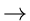B associates buckets (hash values) to keys.

Two main issues:

1.
Collisions

If x1 and x2 are two different keys, it is possible that h(x1) = h(x2). This is called a collision. Collision resolution is the most important issue in hash table implementations.

2.
Hash Functions

Choosing a hash function that minimizes the number of collisions and also hashes uniformly is another critical issue.

Collision Resolution by Chaining

• Put all the elements that hash to the same value in a linked list. See Figure 3.1.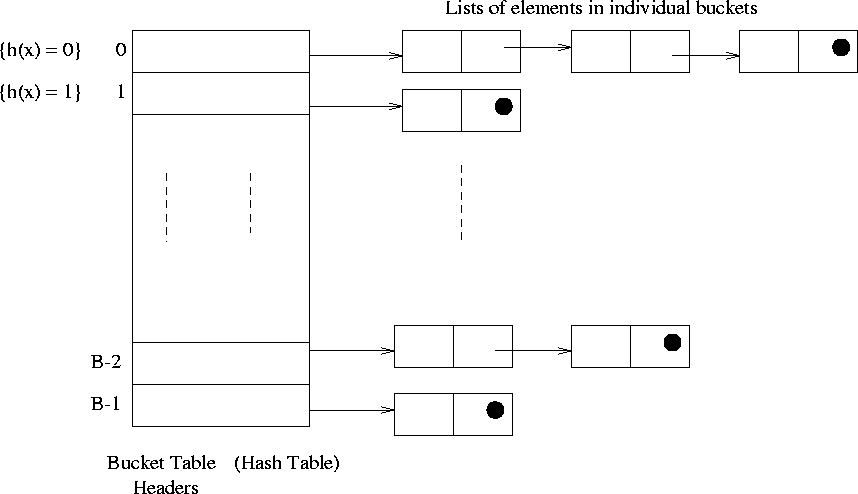Example:

See Figure 3.2. Consider the keys 0, 1, 4, 9, 16, 25, 36, 49, 64, 81, 100. Let the hash function be:

h(x) = x % 7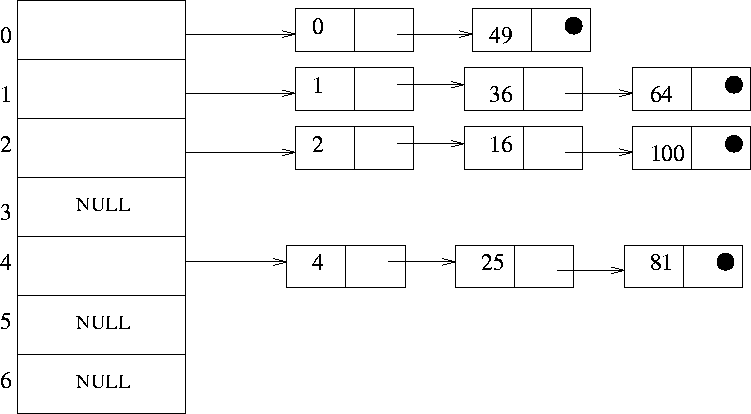• Bucket lists
• unsorted lists
• sorted lists (these are better)

• Insert (x, T)

Insert x at the head of list T[h(key (x))]

• Search (x, T)

Search for an element x in the list T[h(key (x))]

• Delete (x, T)

Delete x from the list T[h(key (x))]

Worst case complexity of all these operations is O(n)

In the average case, the running time is O(1 +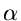), where= load factor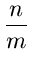(3.1) n = number of elements stored (3.2) m = number of hash values or buckets

It is assumed that the hash value h(k) can be computed in O(1) time. If n is O(m), the average case complexity of these operations becomes O(1) !Next: 3.4 Closed Hashing Up: 3.3 Hash Tables Previous: 3.3 Hash Tables
eEL,CSA_Dept,IISc,Bangalore